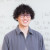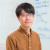Research Fields
Mathematics, Algebraic Geometry
Term and History
2019/04/01 - 2020/09/30 Special Postdoctoral Researcher
2020/10/01 - Visiting Scientist (Main: Assistant Professor, Department of Mathematics, Faculty of Mathematics, Kyushu University)

## Self-introduction

Hello, I am Kenta Sato. My major is mathematics, in particular algebraic geometry. In algebraic geometry, we study algebraic varieties, which are defined as the zero sets of polynomial equations. When you hear polynomials, you may imagine polynomials whose coefficients are integers, rational numbers, or complex numbers. But, it is sometimes important to consider polynomials whose coefficients are in some other field, for example a field of positive characteristic.

I have been studying polynomials, rings and algebraic varieties over a field of positive characteristic. In particular, I am interested in F-singularities and globally F-regular varieties, which are defined in terms of positive characteristic methods. I also hope to find some application of positive characteristic methods to problems in mathematics and other areas.

## Related Events### Math Seminars by Dr. Genki Ouchi and Dr. Kenta Sato

September 24 (Thu) at 16:00 - 18:10, 2020 Seminar iTHEMS Math Seminar### Introduction to Singularity Theory in Algebraic Geometry

May 16 (Thu) at 16:00 - 18:10, 2019 Seminar iTHEMS Math Seminar